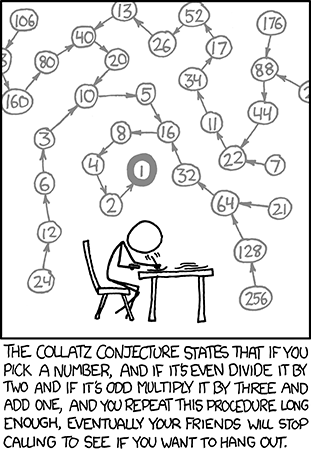You are currently browsing the tag archive for the ‘xkcd’ tag.The following is the first post in 2006 regarding George Vaccaro’s horrifying encounters with the Verizon billing department over the difference between dollars and cents (and other basic math).

Verizon doesn’t know Dollars from Cents

I have a Verizon unlimited data plan in the U.S. and recently crossed the border to Canada. Prior to crossing the border I called customer service to find out what rates I’d be paying for voice and data. The data rate I was quoted was “.002 cents per kilobyte.”

I was surprised at the rate so I confirmed it with the representative I spoke to, and she confirmed it “point zero zero two cents per kilobyte.” I asked her to note that in my account.

I received my bill and was charged $.002/KB – which is dollars – “point zero zero 2 dollars per kilobyte”. As it is translated to cents would be .2 cents or 2 tenths of a cent – which is a 100 times greater rate than I was quoted. My bill for my data usage in Canada was therefore much greater than I had expected – using the quote I was provided before my usage. I have tried to resolve this issue with customer service reps on the phone, but noone seems to see the difference between “.002 cents” and “.002 dollars”. Here is the audio of my most recent call with them on the matter. Original Full Length Recording I started recording when they put on the supervisor – I was a bit ticked at that point. Who knew what confusion “$1 = 100 cents” could cause?

I’m still currently on the hook for the \$71 and change. Hopefully someone at Verizon will figure this out and make ammends.

This is from XKCD, in response to George Vaccaro’s encounters:Here is the timeline of events

TimelineEuler Formula

The Euler formula, sometimes also called the Euler identity, states$\displaystyle e^{ix}=cosx+isinx,$

where${i}$ is the imaginary unit. Note that Euler’s polyhedral formula is sometimes also called the Euler formula, as is the Euler curvature formula.

The special case of the formula with${x=\pi}$ gives the beautiful identity$\displaystyle e^{i\pi}=-1,$

an equation connecting the fundamental numbers${i,\pi,e,1,}$ and${0}$, the fundamental operations${+}$,${\times}$, and exponentiation, the most important relation${=}$, and nothing else. Gauss is reported to have commented that if this formula was not immediately obvious, the reader would never be a first-class mathematician.